【统计学论文】

## 数据挖掘论文范文

### Short-Term Forecast of Foreign Exchange Reserves using Time Serize Analysis with R来源：人大经济论坛论文库　作者：Kaiqing Fan　时间：2016-05-25

Short-Term Forecast of the Monthly Foreign Exchange Reserves

(From 1/2011 to 1/2012)

Kaiqing Fan

Data description:

The monthly foreign exchange reserves for China has been kept going up from 1978, but the available time series data I can find online is only from December 1999. At the end of 1999, Chinese Government decided to improve the number of foreign exchange reserves to prevent the international financial and economic risks. From that time on, the reserves have gone up very quickly. In December 1999, Chinese foreign exchange reserves is only 1546.75 (unit: 100 million US dollars), at the end of 2010, the reserves reached at 28473.38 million US dollars. I choose the monthly foreign exchange reserves for Chinese Government from December 1999 to December 2010 to do time series analysis.

Resource:

http://www.safe.gov.cn/  ,there is an English version in this website.

Introduction

To apply for entering World Trade Organization, Chinese Government adjusted her policies to prepare this application. One of these adjustments was to improve the foreign exchange reserves against the international economic and financial risks. So from December 1999 on, China’s foreign exchange reserves has been going up. From 2004 on, Chinese Government adjusted her policies to appreciate the value of RMB---Chinese dollar by small amplitude, a safe and healthy, and under control steps, lots of US dollars entered into China to arbitrage, this speeded up the accumulation of China’s foreign.

Analysis of the Original Data

library(TSA)

x=scan(file.choose()) ; y=ts(x)

plot(y)

acf(y)

pacf(y)

Pic 1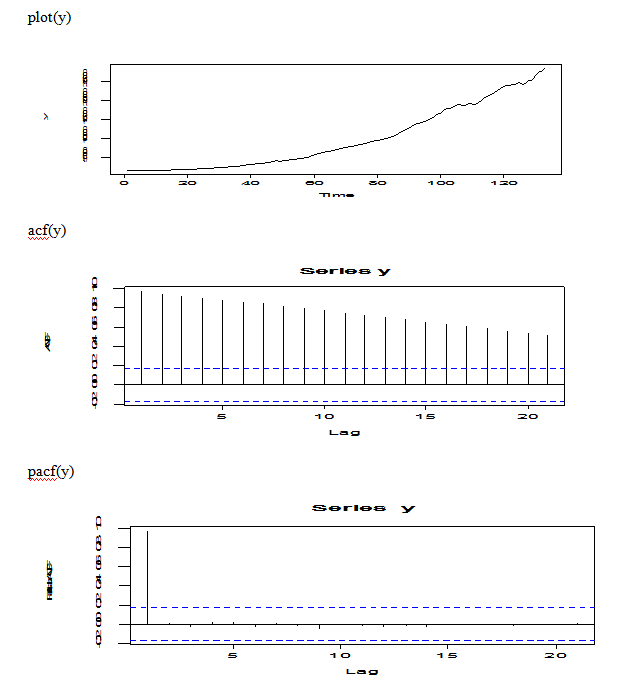From the plot of original data, we may try to do some transformation because the original data is non-stationary and the plot shows increasing trend. From the ACF(y), there is an exp decay, but the plot is dying of very slowly; From the PACF(y), there is 0 for k>1.

Transformations and Candidate Model Identifying

plot(log(y))

Pic 2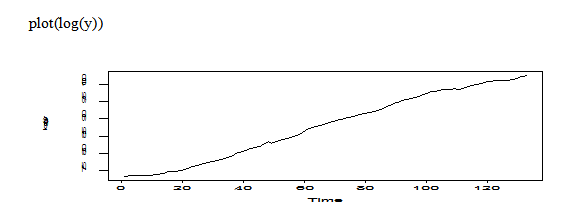The transformation of log original data still shows trend, non-stationary.

plot(diff(y))

Pic 3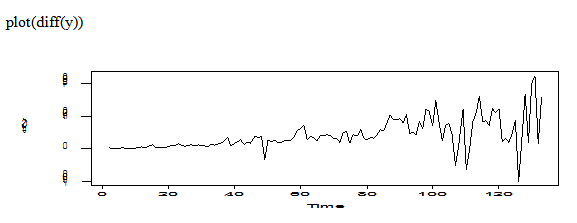ff=diff(x)

Augmented Dickey-Fuller Test

data:  ff

Dickey-Fuller = -3.0121, Lag order = 5, p-value = 0.1544

alternative hypothesis: stationary

The transformation of difference on original data still shows non-stationary. Let’s try difference of log original data.

plot(diff(log(y)))

Pic 4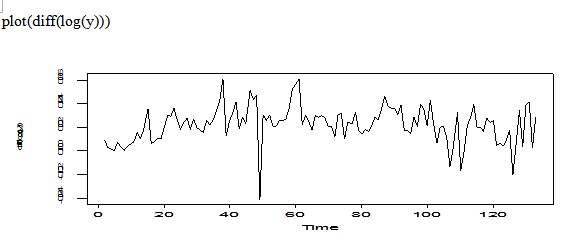fan=diff(log(y))

Augmented Dickey-Fuller Test

data:  fan

Dickey-Fuller = -3.4266, Lag order = 5, p-value = 0.05267

alternative hypothesis: stationary

The transformation of diff(log(fan)) is not too bad, we can think it is stationary.

acf(diff(log(y)))

pacf(diff(log(y)))

Pic 5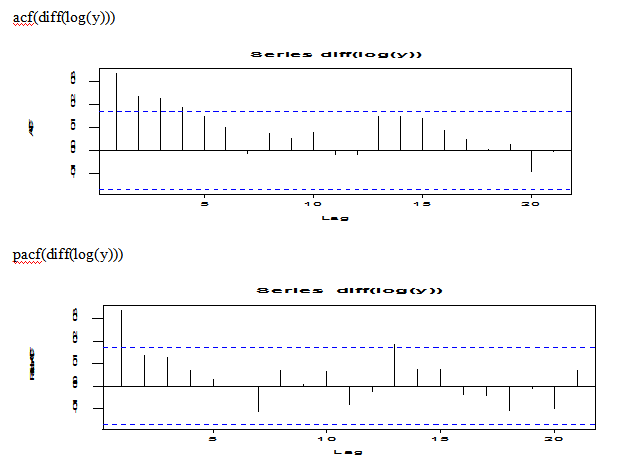The plot of diff(log(y)) looks no trend; the plot of ACF(diff(log(y))) shows some form exp decay; the plot of PACF(diff(log(y))) shows 1 spike. So we may try AR(1) model for the diff(log(y)) transformation.

But this is not much help, we may try looking at EACF.

eacf(diff(log(y)))

AR/MA

0 1 2 3 4 5 6 7 8 9 10 11 12 13

0 x x x x o o o o o o o  o  o  o

1 x o o o o o o o o o o  o  o  o

2 x x o o o o o o o o o  o  o  o

3 x o o o o o o o o o o  o  o  o

4 x o o o o o o o o o o  o  o  o

5 x o o o o o o o o o o  o  o  o

6 o x x o o o o o o o o  o  o  o

7 x x x o x o o o o o o  o  o  o

Let’s try ARMA(1,1), MA(4) model for this diff(log(y)) transformation.

Model Selection by AIC, AICc, BIC and their Coefficients

MA(4) model for diff(log(y))

fan=diff(log(y))

arima(fan,order=c(0,0,4))

Call: arima(x = fan, order = c(0, 0, 4))

Coefficients:           ma1     ma2     ma3     ma4  intercept

0.2551  0.1246  0.1788  0.1482     0.0220

s.e.  0.0868  0.0876  0.0872  0.0870     0.0021

sigma^2 estimated as 0.0002006:  log likelihood = 374.53,  aic = -739.06

IC=function(AIC,n,k){

AICc=AIC+2*k*(k+1)/(n-k-1)

BIC=AIC-2*k+log(n)*k

return(list(c("AIC=",AIC),c("AICc=",AICc),c("BIC=",BIC)))

}

IC(-739.06,length(fan),9)

 "AIC="    "-739.06"

 "AICc="             "-737.584590163934"

 "BIC="              "-713.114782696723"

ARMA(1,1) Model for diff(log(y))

arima(fan, order=c(1,0,1))

Call: arima(x = fan, order = c(1, 0, 1))

Coefficients:           ar1      ma1  intercept

0.8180  -0.5746     0.0217

s.e.  0.1177   0.1667     0.0028

sigma^2 estimated as 0.0001985:  log likelihood = 375.2,  aic = -744.41

IC(-744.41,length(fan),9)

 "AIC="    "-744.41"

 "AICc="             "-742.934590163934"

 "BIC="              "-718.464782696723"

AR(1) Model for diff(log(y))

> arima(fan, order=c(1,0,0))

Call: arima(x = fan, order = c(1, 0, 0))

Coefficients:            ar1  intercept

0.3364     0.0220

s.e.  0.0818     0.0019

sigma^2 estimated as 0.0002069:  log likelihood = 372.52,  aic = -741.05

IC(-741.05,length(fan),9)

 "AIC="    "-741.05"

 "AICc="             "-739.574590163934"

 "BIC="              "-715.104782696723"

Summary of the Comparisons Among the Three Candidate Models For diff(log(y))

 MA(4) model ARMA(1,1) model AR(1) model AIC -739.06 -744.41 -741.05 AICc -737.584590163934 -742.934590163934 -739.574590163934 BIC -713.114782696723 -718.464782696723 -715.104782696723 Coeff ma1     ma2     ma3    ma4  intercept 0.255  0.1246  0.1788  0.1482 0.022 ar1   ma1    intercept 0.818 -0.5746  0.0217 ar1  intercept 0.3364     0.0220

For MA(4) model, we can see that its AIC, AICc, BIC are the largest among the three candidate models, and its coefficients of ma2, ma3 and ma4 are borderline;

For ARMA(1,1) model, its AIC, AICc, BIC are the smallest among the three candidate models, and its coefficients are all OK;

For AR(1) model, its AIC, AICc, BIC are the 2nd smallest among the three candidate models, but its coefficient of ar1, ar3 is pretty good;

So we choose ARMA(1,1) as the best model.

Diagnosis for ARMA(1,1) model of diff(log(y))

mod1=arima(fan, order=c(1,0,1))

tsdiag(mod1)

Pic 6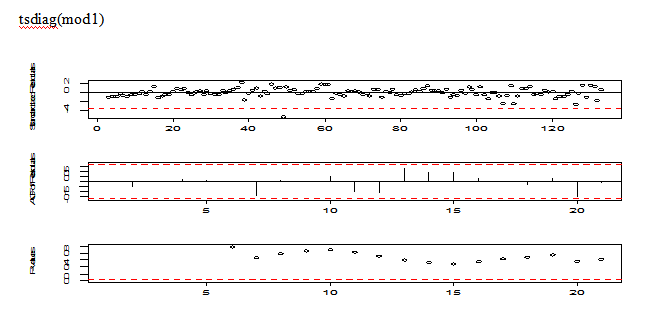The stardandized residuals looks like randomly distributed, but there is one outliers located at around the 50th position; the residual autocorrections look excellent; the plot of p-values are all non-significant.

qqnorm(mod1\$residuals)

abline(mean(mod1\$residuals),sd(mod1\$residuals))

Pic 7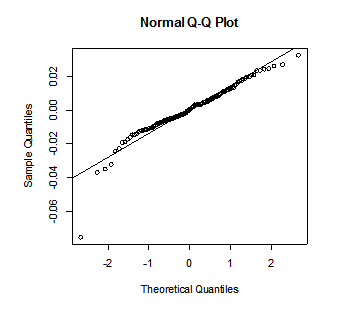QQ plot for normality shows there may one outliers at the left bottom corner, this outlier violates the assumptions of the white noise. We should handle with this potent outlier in the coming future.

res=rstandard(mod1)

plot(res,ylab='ARMA(1,1), residuals')

abline(h=0)

Pic 8If we ignore the point located at around time 50,then the plot of ARMA(1,1) for residuals for diff(log(y)) looks good, there is no trend.

All in all, the model ARMA(1,1) is not as perfect as we expected because of the outlier, we need to deal with it.

Test for outliers and refit the ARMA(1,1) model

detectAO(mod1)

[,1]

ind     48.000000

lambda2 -6.030457

Let’s refit the ARMA(1,1) model incorporating an AO outlier at time t=48. ( t=48 here because this data is diff(log(y)))

If I don’t take differencing, then the outlier would be t-=49, let’s see the following:

library(TSA)

originalx=scan(file.choose())

y=ts(originalx)

y133=window(y, end=133)

rey=ts(y133,start=c(1999,12),frequency=12)

mod1=arima(x=log(rey), order=c(1,1,1),xreg=seq(1,length(rey)))

Call: arima(x = log(rey), order = c(1, 1, 1), xreg = seq(1, length(rey)))

Coefficients:         ar1      ma1    xreg

0.8183  -0.5750  0.0217

s.e.  0.1176   0.1666  0.0028

sigma^2 estimated as 0.0001985:  log likelihood = 375.2,  aic = -744.41

detectAO(mod1)

[,1]

ind     49.000000

lambda2 -6.043367

tg=seq(rey)

AO49=as.numeric(tg==49)

mod2<-arimax(log(rey),xreg=data.frame(tg,AO49),order=c(1,1,1) xreg = data.frame(tg, AO49))

Call: arimax(x = log(rey), order = c(1, 1, 1), xreg = data.frame(tg, AO49))

Coefficients:           ar1      ma1      tg     AO49

0.9772  -0.6707  0.0445  -0.0370

s.e.     NaN   0.0989     NaN   0.0088

sigma^2 estimated as 0.0001834:  log likelihood = 379.78,  aic = -751.56

Warning message: In sqrt(diag(x\$var.coef)) : NaNs produced

tsdiag(mod2)

Pic 9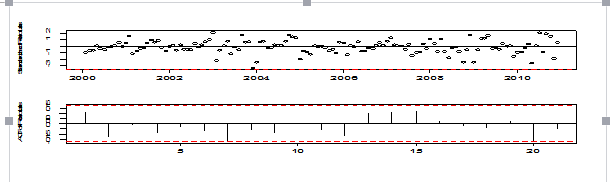Looks like mod2 is not a good model because of R package crushed. We may think of the second best model ARIMA(1,1,0), i.e., AR(1) model on diff(log(rey)).

Let’s try to Test for outliers and refit the ARIMA(1,1,0) model

mod3=arima(x=log(rey),order=c(1,1,0),xreg=seq(1,length(rey)))

Call: arima(x = log(rey), order = c(1, 1, 0), xreg = seq(1, length(rey)))

Coefficients:          ar1    xreg

0.3364  0.0220

s.e.  0.0818  0.0019

sigma^2 estimated as 0.0002069:  log likelihood = 372.52,  aic = -741.05

tsdiag(mod3)

Pic 10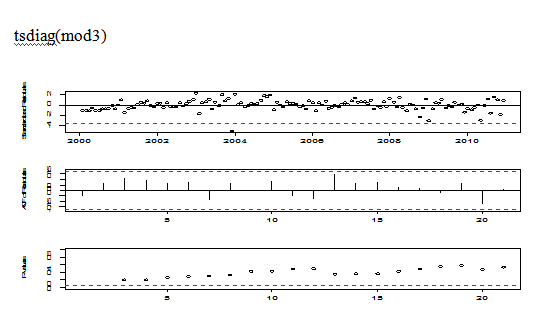The plot of diagnosis is good except for an outlier at around t=49.

qqnorm(mod3\$residuals)

abline(mean(mod3\$residuals),sd(mod3\$residuals))

Pic 11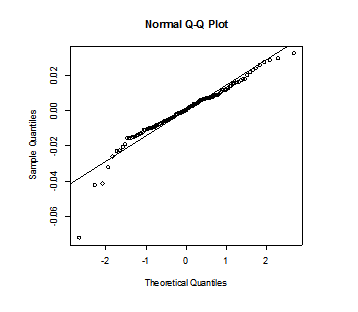QQ plot looks not so nice because of outlier.

detectAO(mod3)

[,1]

ind     49.000000

lambda2 -5.924306

detectIO(mod3)

[,1]

ind     49.000000

lambda1 -5.487006

tg=seq(rey)

AO49=as.numeric(tg==49)

mod4<-arimax(log(rey),xreg=data.frame(tg,AO49),order=c(1,1,0))

Call: arimax(x = log(rey), order = c(1, 1, 0), xreg = data.frame(tg, AO49))

Coefficients:          ar1      tg     AO49

0.4428  0.0223  -0.0389---are very small, non-significant.

s.e.  0.0779  0.0020   0.0079

sigma^2 estimated as 0.0001719:  log likelihood = 384.7,  aic = -763.41

The coefficients of AO49 and tg are all non-significant, so we can ignore tg and AO49 terms,then I do not want to use ARIMA(1,1,0) with AO49 to do forecasting.

So I still choose the model ARIMA(1,1,1) without AO49 to do forecasting.

Rethink the outlier at the 49th position

Let’s take a look at part of the log(rey) and part of original data.

 Time t 47th 48th 49th 50th 51st Month Oct. 2003 Nov 2003 Dec 2003 Jan 2004 Feb 2004 Log(rey) 8.296527 8.343699 8.302144 8.332597 8.358523 Real value 4009.92 4203.61 4032.51 4157.20 4266.39

When we compare the log(rey) values and the real values , we can see that the 49th position is December 2003,its value is 8.302144 , its real number is 4032.51;in November 2003, it is 8.343699,the real number is 4203.61; in January 2004, it is 8.332597, its real number is 4157.20.  They don’t differentiate too much.

The reasons for the decreasing of US dollars on China’s foreign exchange reserves in December 2003 were that the value of US dollars fell after 2003, China’s foreign exchange reserves in the book were serious devaluation, a loss of about \$ 200 billion in the end of 2003. We can think we should keep this outlier in out model, because the depreciation and appreciation of international monetary always happen.

So I may keep this outlier at t=49, then use mod1 ARIMA(1,1,1)to do further forecasting.

Forecasting using mod1 ARIMA(1,1,1)

Pic 12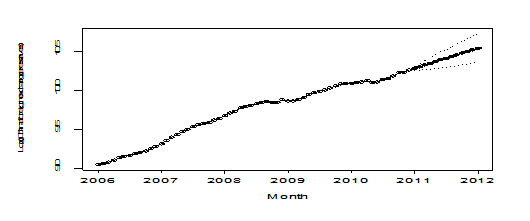The plot of forecast looks very nice.

points(new.t[134:146], rey[134:146], pch=6)

Pic 13The plot of forecast looks very nice.

forecast=result\$pred

actual=y[134:146]

lower=result\$lpi

upper=result\$upi

cbind(lower,forecast,actual,upper)

 month year lower forecast acutal upper Jan 2011 28322.46 29115.53 29316.74 29930.8 Feb 2011 28485.69 29768.92 29913.86 31109.96 Mar 2011 28679.17 30434.27 30446.74 32296.79 Apr 2011 28889.65 31112.23 31458.43 33505.8 May 2011 29115.3 31803.4 31659.97 34739.67 Jun 2011 29356.27 32508.33 31974.91 35998.83 Jul 2011 29613.03 33227.56 32452.83 37283.28 Aug 2011 29885.92 33961.6 32624.99 38593.11 Sep 2011 30175.09 34710.93 32016.83 39928.6 Oct 2011 30480.55 35476.02 32737.96 41290.2 Nov 2011 30802.19 36257.32 32209.07 42678.57 Dec 2011 31139.84 37055.29 31811.48 44094.45 Jan 2012 31493.28 37870.36 上一篇：【数据挖掘算法工程师】国内某上市企业聘 下一篇：Statistical prediction of EI Nino and Hurricanes本论坛由中国人民大学经济学院承办。为做大做强论坛，本站接受风险投资商咨询，请联系（010-62719935） 京ICP备11001960号 京ICP证090565号 京公网安备1101084107号 论坛法律顾问：王进律师 知识产权保护声明 免责及隐私声明 联系QQ：75102711 MSN：pinggu.org@hotmail.com 邮箱：service@pinggu.org 合作咨询电话：(010)62719935 广告合作电话： 13661292478 （刘老师） 投诉电话：(010)68466864 不良信息处理电话：13661292478